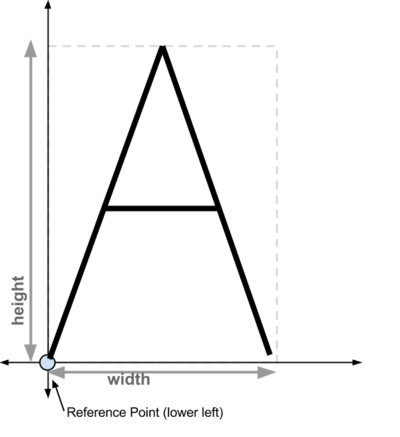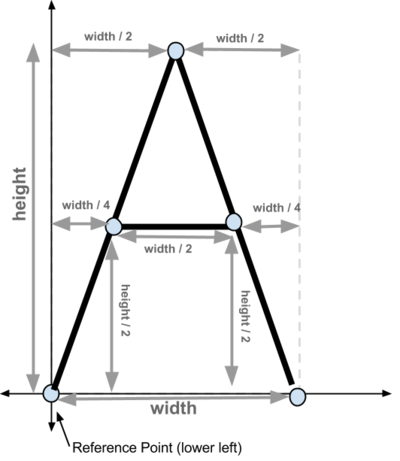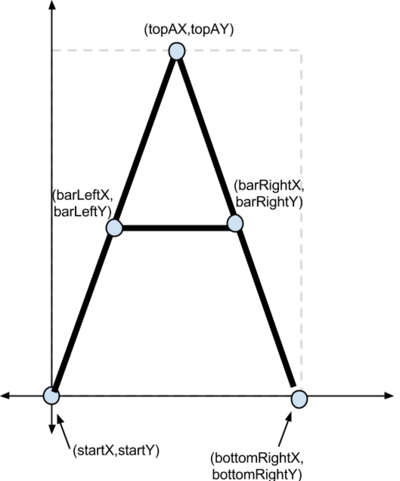# Turtle Letters: 02

Drawing an A

Part of a series of tutorial articles about drawing symbols with turtle graphics.

Code examples referred to on this page can be found here: https://github.com/ucsb-cs8-m17/Lecture3_0810

Instead of using `forward()` and `backward()`, another way to draw with turtle graphics is to calculate the exact points that make up the thing we want to draw, then just connect the dots.

The following code creates a turtle called `someOlTurtle`:

``````import turtle

someOlTurtle = turtle.Turtle()
``````

The following code can then be used to determine the current location of someOlTurtle. At the start of a program, that’s always `(0,0)`, but later in a program, our turtle could be anywhere.

``````startX = someOlTurtle.xcor()
startY = someOlTurtle.ycor()
``````
A in bounding boxSetting `startx` and `starty` to the place the turtle starts out gives us a reference point for our letter. We’ll assume that reference point is at the lower left of the letter we want to draw. So for example, we’ll assume that if we want to draw a `A` with a given width and height, that it is going to be in a so-called “bounding box”, with the reference point at the lower left, as shown in the picture at right labelled “A in bounding box”.

A in bounding box with distancesWe can then compute the vertical and horizontal distances to the various points that make up the lines that we’ll use to draw the A, as shown here in the box at right labelled “A in bounding box with distances”.

A in bounding box with pointsWe can then label all the points as shown in the box at right labelled “A in bounding box with points”.

It’s helpful to give the x and y coordinates of each of the points a name, as shown in the diagram “A in bounding box with points”.

By putting that altogether, we can, at last erite Python code to calculate the x and y values for those points in terms of the values `startX`, `startY`, `width` and `height`:

``````topAX = startX + (width/2)
topAY = startY + height

bottomRightX = startX + width
bottomRightY = startY

barLeftX = startX + width/4
barLeftY = startY + height/2

barRightX = startX + (width/4) + (width/2)
barRightY = startY + height/2
``````

That gives us a way to draw the whole A, as shown here:

``````import turtle

someOlTurtle = turtle.Turtle()

def drawA(width, height):
"""
someOlTurtle will draw the letter A with a given width and height,
with the current location being the lower left corner of the A.
"""

# figure out where we are

startX = someOlTurtle.xcor()
startY = someOlTurtle.ycor()

# figure out the other points using only what we know,
# which is width, height, startX and startY

topAX = startX + (width/2)
topAY = startY + height

bottomRightX = startX + width
bottomRightY = startY

barLeftX = startX + width/4
barLeftY = startY + height/2

barRightX = startX + (width/4) + (width/2)
barRightY = startY + height/2

# draw left hand side of the A
someOlTurtle.goto(topAX,topAY)

# draw the right side of the A

someOlTurtle.goto(bottomRightX, bottomRightY)

# draw bar across the middle

someOlTurtle.up()
someOlTurtle.goto(barLeftX,barLeftY)
someOlTurtle.down()
someOlTurtle.goto(barRightX,barRightY)

# leave turtle at lower right hand corner of letter

someOlTurtle.up()
someOlTurtle.goto(bottomRightX,bottomRightY)
someOlTurtle.down()
``````

You’ll notice that we left the turtle facing right at the right hand corner of the letter. That’s helpful in terms of being able to draw a series of letters going left to right.

## Turtle Graphics letters tutorials: table of contents

Section Code
(github repo)
Topics Covered
Turtle Letters: 01 code Draw a T
Turtle Letters: 02 code Drawing an A
Turtle Letters: 03 code The bounding box, reference point, and Drawing an O (or a 0)
Turtle Letters: 04   Planning your letters inside a bounding box (planning P and C)